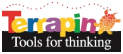Terrapin ResourcesThe odds of two people in a room not having the same birthday (assuming birthdays fall equally on all days of the year and that there are 365 days in the year) is 364/365 = .997 or 99.7%. If another person enters the room, he or she has to avoid two dates, the first person’s birthday which he does with odds of 364/365 and then there are only 363 days he has to avoid to not have the same date as the second person, so those odds are 363/365. Therefore, the odds of that third person in the room not having the same birthday as the other two is 364/365 times 363/365 = .992 or 99.2%.

We can keep doing this until we get odds of less that 50% that the nth person entering the room will not have the same birthday as any other person in the room. To do the opposite: finding out if two people in a room have the same birthday, subtract not having the same birthday from 1, since 1 is the probability of everything, i.e. the “universe”, of either having the same birthday or not having he same birthdy.

So put in a number less than 50 in the SAMEBIRTHDAY procedure, for example SAMEBIRTHDAY 12, and the program will spit out the odds of two people having the same birthday in a room with 12 people.

Find out how many people need to be in a room to have a better than 50% chance of two of them having the same birthday!

#### SAMEBIRTHDAY.lgo

``````  TO SameBirthday :number
IF :number > 50 [
PR "|Large numbers of people approach 100% probility that two will have the same birthday but never quite get to 100% because there is always a chance that two people will not have the same birthday no matter how large the group|
STOP
]
IF :number < 2 [
PR "|There must at least be 2 people in the room to make a comparison|
STOP
]
; calculate the probability factor by counting down from 364 to (366 - number)
; use 366 because the number starts at 2
LMAKE "factor 1
FOR (LIST "N 364 (366 - :number) -1) [
LMAKE "factor :factor * (:N / 365)
]
(PR "|If| :number "|people are in the same room, there is a probability of| 100 * (1 - :factor) "|% that two people will have the same birthday|)
END
``````

 Procedure SAMEBIRTHDAY Description Calculate the probability of two people having the same birthday Level Beginner Tags Math, Birthday